## NLPS Learns

Generic filters
Filter by Subject
Newsletter
Elementary Newsletter
Secondary Newsletter
Literacy
Reading
Writing
Numeracy
Computational Fluency
Number Sense
French Immersion
Educational Technology
ADST
Google
Content Creation
Science
Social Studies
BAA Courses
Course Outlines
Physical and Health Education
Sexual Health
Mental Health
ELL
Indigenous Understandings
Hul̓q̓umín̓um̓
Reconciliation
Environmental Stewardship
Outdoor Education
Reporting
Elementary Electronic Report Card
MyEdBC
PLC
Collaborative Inquiry
Social Justice
Inclusion
Anti-Racism and Cultural Safety
SOGI
/ / / Numeracy Planning

# Numeracy

## Lesson PlanningIdeas

What could your lesson look like?  Are you looking for a structure that allows you to integrate rich instructional routines that naturally differentiate and help students develop their core and curricular competencies?  The following lesson structure is just one example of how this can be done.

## Number Talks- Equations or strings

Number talks equations or strings are used to provide students with regular computation practice while developing mental math strategies.  A problem or a string of connected problems are presented to the students on the board and the students are asked to find an answer and be be prepared to share their strategy.

Why use Number Talks?

• Provides multiple entry points and honors multiple thinking strategies
• Develop mental math strategies
• Explain and justify mathematical ideas and decisions
• Engage all students in mathematical conversationsRachel Lambert

## Guiding Questions

• What answers did you get?
• What strategies did you use?
• Is there another way to thikn about this problem?
• Can you connect _____ strategy to _____.

## Strategies for Solving

Possible strategies when students are prompted with 5 x 18.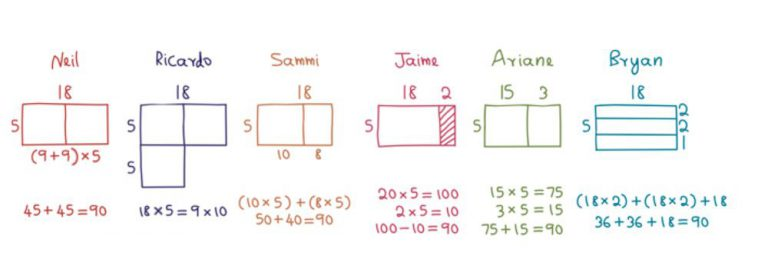## Number Talk Images

Number talk images are similar to a number talk where you use an image to drive conversation around finding a quantity.

Why use Number Talk Images?

• Provides multiple entry points and honors multiple thinking strategies
• Use and develop mathematical vocabulary
• Develop conceptual understanding through visualizing math concepts
• Explain and justify mathematical ideas and decisions
• Engage all students in mathematical conversations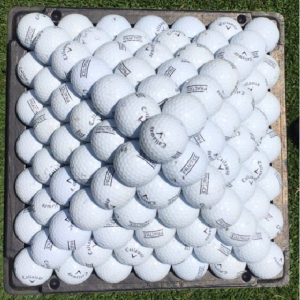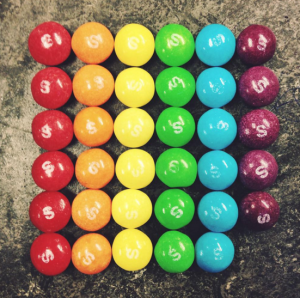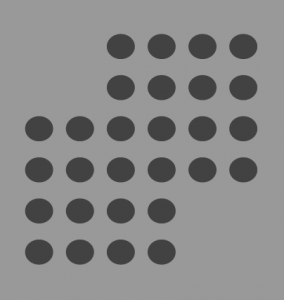## Guiding Questions

• What do you notice?
• What do you wonder?
• How many?
• How do you see them?
• Can you see them in another way?

## Tips for Using Number Talk Images

• Focus on the quantity and how it is displayed in the image
• Annotate to make student thinking visible ( connect pictorial to symbolic forms)
• Project on a white board or print multiple copies of image for annotation
##### Number Talk Images Website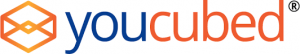## Fraction Talks

A fraction talk is a number talk that only focuses on fractions and fractional thinking.  An image is displayed that shows fractional relationships.  Students then need to justify and which fraction that they see.

Why?

• Communicate mathematical thinking and reasoning in many ways about fractions
• Use and develop mathematical vocabulary
• Develop conceptual understanding of fractions
• Connect mathematical concepts around fractions
• Allows student to visually compare, find equivalent, add, subtract, multiply or divide fractions
• Engage all students in mathematical conversations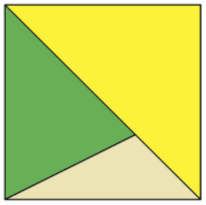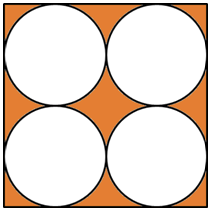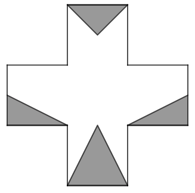## Guiding Questions

• What fraction do you see?
• How do you see the fraction?
• Can you find all other sections that are the same size as _____?
• Can you find all sections that represent _____?
• If the shaded section is worth _____, how big is each other section?
• What other fractions do you see?
• What needs to be added to make the shaded section equal to _____?

## Tips for Using Fraction Talks

• Choose rich sets to build conceptual understanding
• Accept all answers
• Introduce vocabulary when students need it
• Trust students to build connections
•  Summarize student thinking before moving to next student
• Let students turn and talk before sharing thinking
• Have students build there own set or finish a set
• Provide graph paper to sketch their ideas
• Encourage the use of unit fractions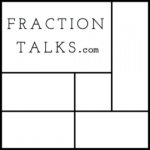##### Fractions Talks Website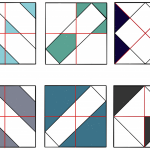## Which One DoesN't Belong?

In this routine, students will will be presented with 4 images and the will have to determine how they are all unique and how they are all the same.   Allow the students to interact with the images how they choose there is not one right answer and their answers may not be very ‘mathy’ but you will start to hear all of your students voices!

Why?

• Communicate mathematical thinking and reasoning in many ways
• Use and develop mathematical vocabulary
• Connect mathematical concepts
• Engage all students in mathematical conversations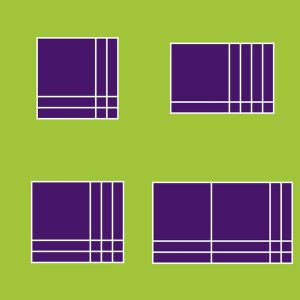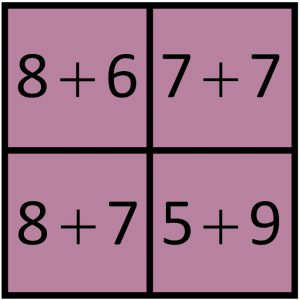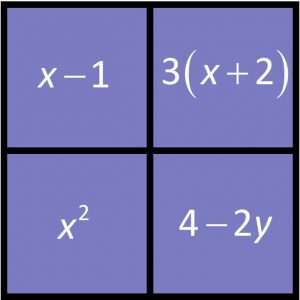## Guiding Questions

• What do you notice?
• What are they all alike?
• How are they all different?
• Which One Doesn’t Belong?
• Can you share your reasoning?

## Tips for Using WODB

• Choose rich sets to build conceptual understanding
• Accept all answers
• Introduce vocabulary when students need it
• Trust students to build connections
•  Summarize student thinking before moving to next student
• Let students turn and talk before sharing thinking
• Have students build there own set or finish a set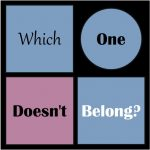## Estimation Tasks

Begin by showing students an image of a quantity.  Then, together as a class  determine an estimate that is reasonably too high and reasonably to low.  Have the students think about their best estimates and how they could represent their reasoning.

Why Use Estimation Routines?

•  Engage students in ordering, comparing including benchmarks
• Improve students spatial reasoning
• Develop students number sense
• Develop the skills to defend the reasonableness of an answer
##### Possible Prompts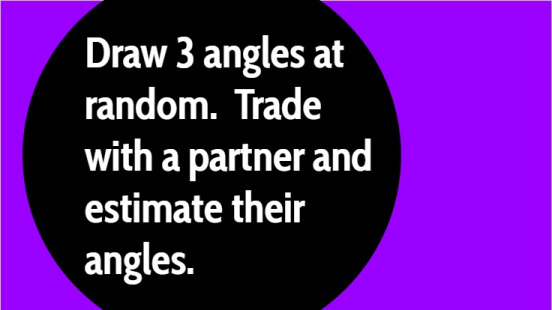Mark Chubb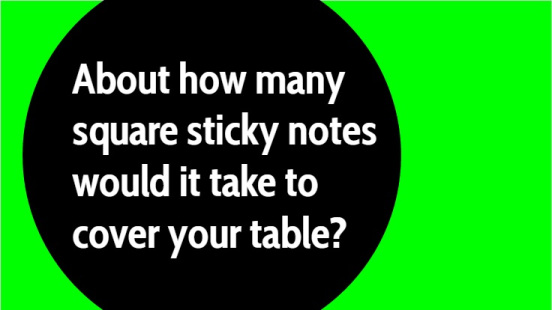## Guiding Questions

• What is a reasonably too low estimate?
• What is a reasonably too high estimate?
• What is a reasonable estimate?
• Can you connect _____ strategy to _____.

## Estimation prompts

The following sites have great visual estimations for use in you classroom.